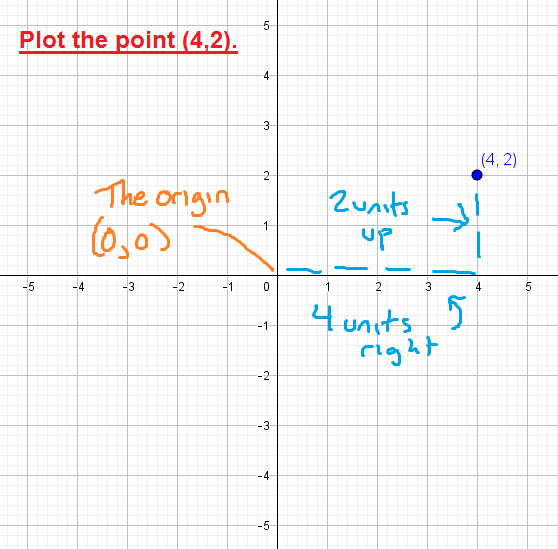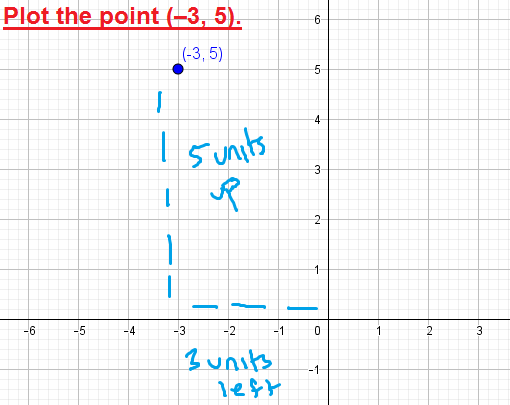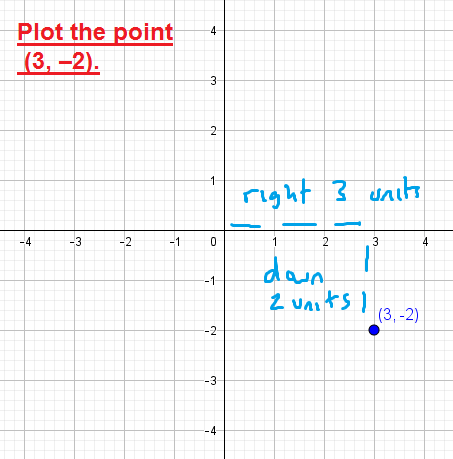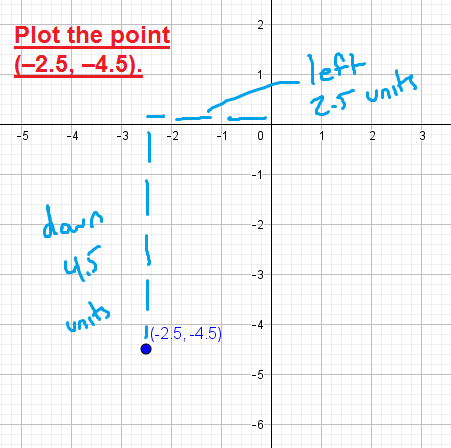﻿ Plotting points (ordered pairs) in the coordinate plane - MathBootCamps

# Plotting points (ordered pairs) in the coordinate plane

To plot a point in the xy-coordinate plane (also called the Cartesian coordinate plane), you just have to know how to read the values or numbers in the ordered pair that defines the point. In this lesson, we will look at exactly how to do this!

Each number in an ordered pair is called a “coordinate”. The first number is called the “x-coordinate” and the second number is called the “y-coordinate”. These two numbers tell you in which part of the coordinate plane your point lies.## Examples

To plot a point, start at the origin, which is the center of the graph (the point (0,0)). Then, the x-coordinate will tell you how many units to move left or right, and the y-coordinate will tell you how many units to move up or down.If the x-coordinate is negative, then you will move left instead of right.It is the same idea with a negative y-coordinate. You will move down from the origin instead of up.## What if the coordinates are fractions or decimals?

The coordinates in an ordered pair can be any real number. This means that not only can they be positive or negative, but they can also be fractions or decimals. Even in this case, the same general idea applies. But, depending on the decimal or fraction, you might have to estimate the location of the point a little bit.## Summary

A point is made up of two coordinates: the x-coordinate and the y-coordinate. Each one gives you a piece of information you can use to plot the point in the coordinate plane.